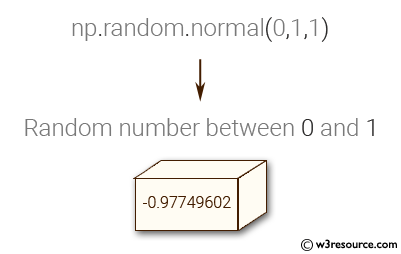﻿ NumPy: Generate a random number between 0 and 1 - w3resource

# NumPy: Generate a random number between 0 and 1

## NumPy: Basic Exercise-17 with Solution

Write a NumPy program to generate a random number between 0 and 1.

Sample Solution :

Python Code :

``````import numpy as np
rand_num = np.random.normal(0,1,1)
print("Random number between 0 and 1:")
print(rand_num)
``````

Sample Output:

```Random number between 0 and 1:
[-1.15262276]
```

Pictorial Presentation:Python Code Editor:

Have another way to solve this solution? Contribute your code (and comments) through Disqus.

What is the difficulty level of this exercise?

﻿

Inviting useful, relevant, well-written and unique guest posts